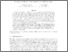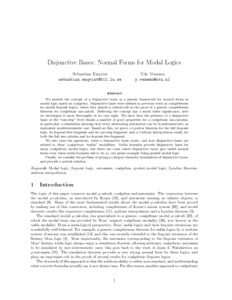# PP-2017-05: Disjunctive bases: normal forms for modal logics

PP-2017-05: Enqvist, Sebastian and Venema, Yde (2017) Disjunctive bases: normal forms for modal logics. [Pre-print] (Submitted)Preview Text disj-bases-report.pdf Download (424kB) | Preview

## Abstract

We present the concept of a disjunctive basis as a generic framework for normal forms in modal logic based on coalgebra. Disjunctive bases were defined in previous work on completeness for modal fixpoint logics, where they played a central role in the proof of a generic completeness theorem for coalgebraic mu-calculi. Believing the concept has a much wider significance, here we investigate it more thoroughly in its own right. We show that the presence of a disjunctive basis at the “one-step” level entails a number of good properties for a coalgebraic mu-calculus, in particular, a simulation showing that every alternating automaton can be transformed into an equivalent nondeterministic one. Based on this, we prove a Lyndon theorem for the full fixpoint logic, its fixpoint-free fragment and its one-step fragment, and a Uniform Interpolation result, for both the full mu-calculus and its fixpoint-free fragment.

We also raise the questions, when a disjunctive basis exists, and how disjunctive bases are related to Moss’ coalgebraic “nabla” modalities. Nabla formulas provide disjunctive bases for many coalgebraic modal logics, but there are cases where disjunctive bases give useful normal forms even when nabla formulas fail to do so, our prime example being graded modal logic.

Finally, we consider the problem of giving a category-theoretic formulation of disjunctive bases, and provide a partial solution.

Item Type: Pre-print PP-2017-05 Prepublication (PP) Series 2017 modal logic, fixpoint logic, automata, coalgebra, graded modal logic, Lyndon theorem, uniform interpolation ComputationLogicMathematics Yde Venema 27 Apr 2017 13:47 27 Apr 2017 13:47 https://eprints.illc.uva.nl/id/eprint/1532View Item